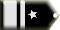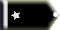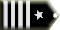# calculation of rate of descentbocboc Guest

Hi, i get a little confused about how the rate of descent is calculated in the flyng lessons

it said that if you want to lose 10,000 feet of descent then
10,000*3=30,000 feet need to go (3:1 rule) BUT it said that 30,000 feet equals to 30 nautical mile (NM)??? uh?? So a nautical mile is equal to 1,000 feet ? I just check it up and its defininately not 1,000 feet.

Then it said that you have to multiply the ground distance in KTS (what is that, knots per hour???) it used an example value of 400 knots, by 6 to get the rate of descent of 2400 feet per minutes . How did it get a unit knots per hour to a feet per minute by multiplying by 6? Is there some unit conversion?CRJCapt Chief Captain

Distance required to descend

10,000 ft. descent

Altitude( in thousands) X 3= distance to start descent

10X3= 30 nautical miles

Rate of descent

1/2 of Ground speed in knots

400 knots / 2 = 200 or 2000 fpm

150 knots /2 = 75 or 750 fpm

Nautical mile is 6076 ft. Statue mile is 5280 ft. in length.Guest

okay lets try it out

400 nots is
(1 knot = 0.514444444 m / s)

205.7776 m/s

multiply by 60 s = 12346.656 m/minute
convert it to feet per minute yields 37,038 feet per minute

How did it arrived at the result of 2,400 per minute ??????

it really baffles meGuest

Distance required to descend

10,000 ft. descent

Altitude( in thousands) X 3= distance to start descent

10X3= 30 nautical miles

=========

SO a nautical mile is 1000 feet?????
i check it up
1 nautical mile = 1.85200 kilometers
or 1852 meters---> 5556 feets!

Rate of descent

1/2 of Ground speed in knots

400 knots / 2 = 200 or 2000 fpm

150 knots /2 = 75 or 750 fpm

=======

but a knot is = 0.514444444 m / s

or 0.51 meter/second * 60 second/minute * 3 feet /meter = 91.8 feet/minute

a knot is 91.8 f/minute

or if you do the conversion, you shouold multiply by 91.8 instead of 6???

======

Nautical mile is 6076 ft. Statue mile is 5280 ft. in length.Guest

okay it must take into account that the ground speed is 3 times greater than the descent rate according to the 3:1 rule

so 400 nots =200 m/s = 600ft/s divide by 3 ==> 200 ft/s * 60 seconds ===>12,000 ft/minute

arg still not equal to the 2400 ft/s

is the math complete junk in the flying lessons ? how come Rod completely oblivious about this???antone First Officer

CrjCapt said that:

"Altitude( in thousands) X 3= distance to start descent

10X3= 30 nautical miles"

This is not an assertion that one nm=1000 feet. It is an approximation used to help calculate when the descent should begin - if one is 10,000 altitude, the descent should begin 30nm from, I suppose, the runway threshold.CrashGordon Chief Captain

Anonymous wrote:

okay it must take into account that the ground speed is 3 times greater than the descent rate according to the 3:1 rule

so 400 nots =200 m/s = 600ft/s divide by 3 ==> 200 ft/s * 60 seconds ===>12,000 ft/minute

arg still not equal to the 2400 ft/s

is the math complete junk in the flying lessons ? how come Rod completely oblivious about this???No, the problem is that you are hell-bent on confusing yourself. It is not about converting units of measure. It is about how many feet per minute descent is required to reduce your altitude to ground level in the time it will take you to go from your present position to the runway. The only reason distance to the airport is used is to determine the amount of time you have to descend. After that the equation is solely about altitude divided by time.Jonathan (99jolegg) Chief CaptainGuest

you talk about time but that is what the purpurse of calculating the exact rate of descent needed to take to match the ground speed given the distance it need to cover so that the altitude lost also match the ground distance ran. If there is an inconsistency in the calculation then they both wont meet at the distance and altitude goal at the same time. What is the point then taking an arbitrary value of distance that does not match the altitude and then take a ground speed multiply by a mysterious factor of 6 which is not a conversion factor at all?

And another point i dont understand, why is that the ground speed dont match the airspeed when flying at level say at 30,000 ft, because of the altitude, density of air??? lolllllJonathan (99jolegg) Chief Captain

Anonymous wrote:

you talk about time but that is what the purpurse of calculating the exact rate of descent needed to take to match the ground speed given the distance it need to cover so that the altitude lost also match the ground distance ran. If there is an inconsistency in the calculation then they both wont meet at the distance and altitude goal at the same time. What is the point then taking an arbitrary value of distance that does not match the altitude and then take a ground speed multiply by a mysterious factor of 6 which is not a conversion factor at all?

And another point i dont understand, why is that the ground speed dont match the airspeed when flying at level say at 30,000 ft, because of the altitude, density of air??? lolllll

I really think you are making it a lot more complicated than it is.

Since you like complexity, try this:

1. top of decent = 3 x (altitude - airport elavation) divided by 1000

For EGLL, 3 x (5000 - 80) / 1000
4920 x 3 / 1000 = 14.7nm

2. For the rate of decent you need to no the True Airspeed (TAS)
..... IAS X 0.02 X (Altitude / 1000) + IAS

So.... 300 x 0.02 X 5 + 300 = 330....so 330 is the true airspeed

3. FOR RATE OF DECENT, MULTIPLY TAS BY 5, i.e. 5 X 330 = 1650fpm

At 14.7 nm away from the airport, you need to decend at 1650fpm

You now know the top of descent (TOD) and the vertical descent speed. When you are descending, you need to set the speed dictated by the manual for that aircraft, i.e. around 0.75MACH to FL280 then reduce to 260 KIAS, and then 240 KIAS for FL100.Greg1138 TraineeGuest

========

its not that hard thinking at all, it just it doesnt make sense some of the calculation it uses to get the numbers. If you know a bit of science math, then thats how you perceive. Remember when your teacher said always put a unit when calculatting a value? This is not algebra neither. Its a concrete problem. So when you multiply by a number which has no unit like 6 and get a different unit, you wonder what that 6 represents or any other value used to convert to a different value. But anyway if thats how it work its a guessing game instead of precision, no pun intentedGermán Campopiano (Oberkomando) Captain

Thanks for all guys, i have to check all this again. 👍Guest

its really freaking easy now after reading back the lesson and giving it serious thoughtwhere it got the 6 which is a conversion actually a factor.

So if you want to lose 10,000 feet of altitude you need to go 30 nm times that amount given a rate of 1000 feet of descent to a 1 nm of ground distance ratio.

30 nm= 30* 1856m * 3 ft/m= 167,040ft

167,040ft / 10,000 ft=16.7

the ratio of 10,000 to 30 nm is 16.7 but not 3:1 as i was thinking

and the ground speed is 400KTS (which is a unit of speed in m/s, this is where i got further mixed up)

and for the rate of descent ,you need to divide by 16.6 instead of 3

400knot/16.7 = 23 knots of descent <-- would that make sense

imagine you rate of descent is a whooping 133 knots if it was 3 times slower, at that rate you are litterally in a state of weightlessnessand for the conversion part
23 knots

23knot=23 * 0.5 m/s * 3 ft/m * 60 s/min=2170 f/min

of course all this nonesense is for proving that there is a proportionality in the rate of descent with the ground speed given a constant speed (a 16,7 times factor instead of 3). If you want easy conversion try the easy method that everybody suggested.CrashGordon Chief Captain

Everyone has gone math-happy.

I like to step my descent. When I'm 100 miles out at 10,000 ft, I go to:

5,000 ft AGL at 50 miles out
3,500 ft AGL at 35 miles out
2,000 ft AGL at 20 miles out

From there I am guided by the terrain and even if I have to maintain that altitude until 10 miles out, it only requires a gentle 200 fpm descent after that until I am at the runway.

Notice, I haven't calculated anything. If I can't hit those altitudes by simply looking at my instruments and adjusting accordingly, I should stay on the ground.Jimewaka Guest

The easiest and most acurate way to do it in your head is altitude to lose ie 230*3 so the first two numbers of the altitude you need to lose '23' for 230 (30,000)*3=69 so start decent 69 miles out, but then divide the milage by 3 to get your feet per minute, ie, 69/3=23, so decend at 2300 ft per minute and it'll work out every time with 3 degree decent path.Guest

When using FSX and others the A/C tells you when to descend and to what height if you are given the wrong height 30 miles from touchdown then you will have dive more to make up for the mistakes OR go around and try again OR ignore the a/c and proceed your own way.
It happens to me a lot in FSX and 2004 but not with X-Plane why I wonder.
PC

All times are GMT Page 1 of 1